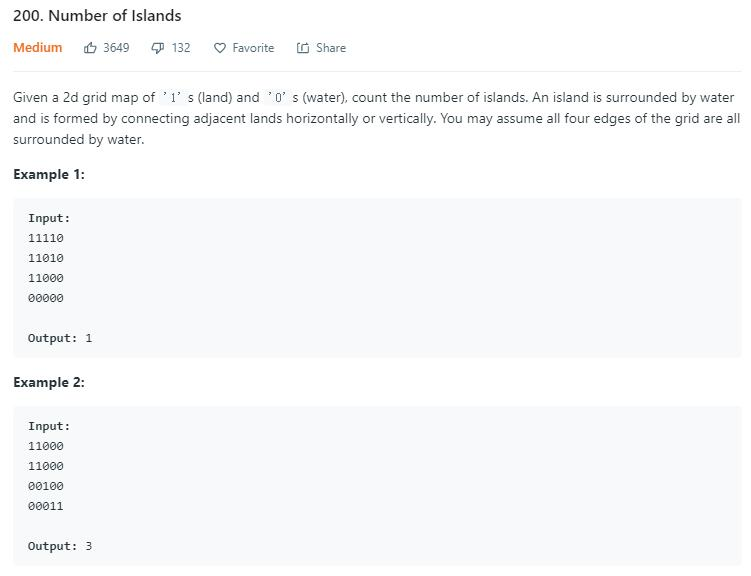# 题目描述（中等难度）# 解法一

 1 0 0 0
1  1 0 0 0
0 0 1 0 0
0 0 0 1 1

2 2 0 0 0
2 2 0 0 0
0 0 1 0 0
0 0 0 1 1

2 2  0  0 0
2 2  0  0 0
0 0  0 0
0 0  0  1 1

2 2 0 0 0
2 2 0 0 0
0 0 2 0 0
0 0 0 1 1

2 2 0  0  0
2 2 0  0  0
0 0 2  0  0
0 0 0  1

2 2 0 0 0
2 2 0 0 0
0 0 2 0 0
0 0 0 2 2



public int numIslands(char[][] grid) {
int count = 0;
int rows = grid.length;
if (rows == 0) {
return 0;
}
int cols = grid.length;
for (int r = 0; r < rows; r++) {
for (int c = 0; c < cols; c++) {
if (grid[r][c] == '1') {
count++;
marked(r, c, rows, cols, grid);
}
}
}
return count;
}

private void marked(int r, int c, int rows, int cols, char[][] grid) {
if (r == -1 || c == -1 || r == rows || c == cols || grid[r][c] != '1') {
return;
}
//当前 1 标记为 2
grid[r][c] = '2';

//向上下左右扩展
marked(r + 1, c, rows, cols, grid);
marked(r, c + 1, rows, cols, grid);
marked(r - 1, c, rows, cols, grid);
marked(r, c - 1, rows, cols, grid);

}


public int numIslands(char[][] grid) {
int count = 0;
int rows = grid.length;
if (rows == 0) {
return 0;
}
int cols = grid.length;
for (int r = 0; r < rows; r++) {
for (int c = 0; c < cols; c++) {
if (grid[r][c] == '1') {
count++;
bfs(r, c, rows, cols, grid);
}
}
}
return count;
}
private void bfs(int r, int c, int rows, int cols, char[][] grid) {
queue.offer(r * cols + c);
while (!queue.isEmpty()) {
int cur = queue.poll();
int row = cur / cols;
int col = cur % cols;
//已经标记过就结束，这句很关键，不然会把一些节点重复加入
if(grid[row][col] == '2'){
continue;
}
grid[row][col] = '2';
//将上下左右连通的 1 加入队列
if (row != (rows - 1) && grid[row + 1][col] == '1') {
queue.offer((row + 1) * cols + col);
}
if (col != (cols - 1) && grid[row][col + 1] == '1') {
queue.offer(row * cols + col + 1);
}
if (row != 0 && grid[row - 1][col] == '1') {
queue.offer((row - 1) * cols + col);
}
if (col != 0 && grid[row][col - 1] == '1') {
queue.offer(row * cols + col - 1);
}

}
}


# 解法二 并查集

• Find：确定元素属于哪一个子集。它可以被用来确定两个元素是否属于同一子集。
• Union：将两个子集合并成同一个集合。

int node(int i, int j) {
return i * cols + j;
}


nums 来记录当前有多少个岛屿，初始化的时候每个 1 都认为是一个岛屿，然后开始合并。

class UnionFind {
int[] parents;
int nums;

public UnionFind(char[][] grid, int rows, int cols) {
nums = 0;
// 记录 1 的个数
for (int i = 0; i < rows; i++) {
for (int j = 0; j < cols; j++) {
if (grid[i][j] == '1') {
nums++;
}
}
}

//每一个类初始化为它本身
int totalNodes = rows * cols;
parents = new int[totalNodes];
for (int i = 0; i < totalNodes; i++) {
parents[i] = i;
}
}

void union(int node1, int node2) {
int root1 = find(node1);
int root2 = find(node2);
//发生合并，nums--
if (root1 != root2) {
parents[root2] = root1;
nums--;
}
}

int find(int node) {
while (parents[node] != node) {
parents[node] = parents[parents[node]];
node = parents[node];
}
return node;
}

int getNums() {
return nums;
}
}

int rows;
int cols;

public int numIslands(char[][] grid) {
if (grid.length == 0)
return 0;

rows = grid.length;
cols = grid.length;
UnionFind uf = new UnionFind(grid, rows, cols);

for (int row = 0; row < rows; row++) {
for (int col = 0; col < cols; col++) {
if (grid[row][col] == '1') {
// 将下边右边的 1 节点和当前节点合并
if (row != (rows - 1) && grid[row + 1][col] == '1') {
uf.union(node(row, col), node(row + 1, col));
}
if (col != (cols - 1) && grid[row][col + 1] == '1') {
uf.union(node(row, col), node(row, col + 1));
}
}
}
}
return uf.getNums();

}

int node(int i, int j) {
return i * cols + j;
}